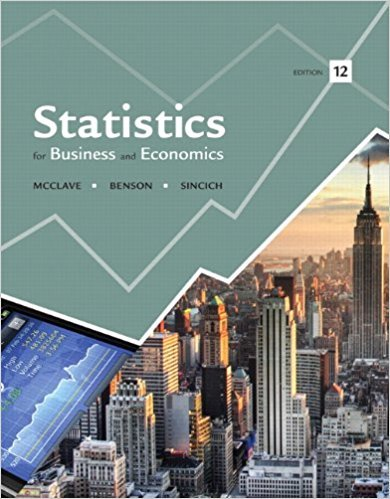×
Get Full Access to Statistics For Business And Economics - 12 Edition - Chapter 5 - Problem 18e
Get Full Access to Statistics For Business And Economics - 12 Edition - Chapter 5 - Problem 18e

×

# A random sample of n = 64 observations is drawn from aISBN: 9780321826237 51

## Solution for problem 18E Chapter 5

Statistics for Business and Economics | 12th Edition

• Textbook Solutions
• 2901 Step-by-step solutions solved by professors and subject experts
• Get 24/7 help from StudySoup virtual teaching assistantsStatistics for Business and Economics | 12th Edition

4 5 1 274 Reviews
29
4
Problem 18E

Problem 18E

A random sample of n = 64 observations is drawn from a population with a mean equal to 20 and a standard deviation equal to 16.

a. Give the mean and standard deviation of the (repeated) sampling distribution of.

b. Describe the shape of the sampling distribution of Does your answer depend on the sample size?

c. Calculate the standard normal z-score corresponding to a value of= 15.5.

d. Calculate the standard normal z-score corresponding to=23

Step-by-Step Solution:

Solution:

Step 1 of 3:

A random sample of n=64 observations is drawn from a population with a mean equal to 20 and the standard deviation equal to 16.

1. We have to find the mean and standard deviation of the sampling distribution of.
2. We have to describe the shape of the sampling distribution.
3. We have to calculate the standard normal Z-score corresponding to a value of=15.5.
4. We have to calculate the standard normal Z-score corresponding to=23.

Step 2 of 3

Step 3 of 3

##### ISBN: 9780321826237

Unlock Textbook Solution